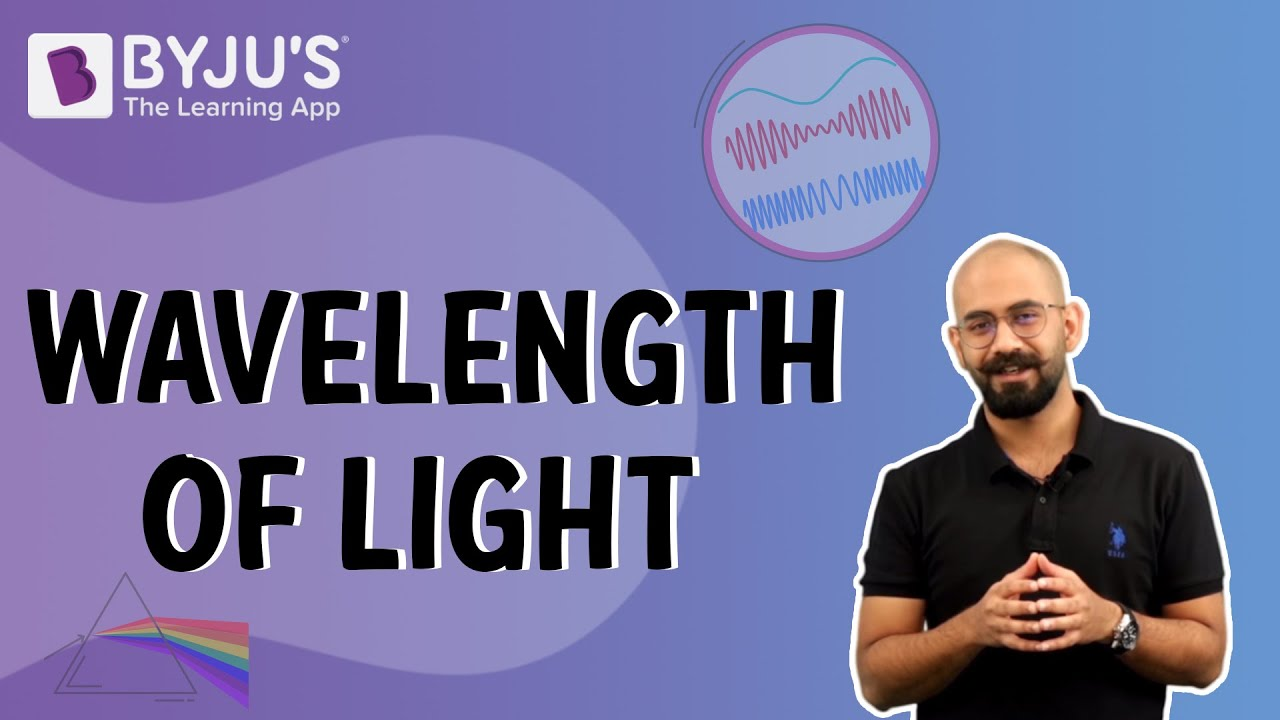Jet Set Go! All about Aeroplanes Jet Set Go! All about Aeroplanes

# Phase Angle

A wave that repeats as a function of time and position is referred to as a periodic wave. Amplitude, frequency, wavelength, speed, and energy describe the properties of the wave. The phase angle is used to describe the characteristics of a periodic wave. Let us learn more about the phase angle in this session.

## What is Phase Angle?

The phase angle is the characteristic of a periodic wave. It is synonymous to Phase in many contexts. In phasors, a wave exhibits twofold characteristics: Magnitude and Phase.

The phase angle refers to the angular component of a periodic wave.

It is a complex entity. A periodic wave is represented as:

$$\begin{array}{l}A\angle \theta\end{array}$$

Where,

• A is the magnitude
• θ is the phase angle

It is measured using degrees or radians

## Measurement of a Phase Angle

Consider a periodic wave. The phase angle can be measured using the following steps:

1. Phase angle can be measured by measuring the number of units of angular measure between the reference point and the point on the wave. The reference point can be on the same wave or another wave.
2. The reference point is chosen from the projection of a rotating vector to the real axis of an Argand diagram.
3. The value of the point on the abscissa corresponding to the point on the wave gives the phase angle of that point.

In general, the wave can be plotted on any standard coordinate system. One complete cycle of the wave has 360º of phase angle in the Cartesian plot.

The phase angle plays a crucial role in electronics where the voltage and various sinusoidal waves are involved. In electronics, the number of electrical degrees of lag or lead between the voltage and current waveforms in an ac circuit is also defined as phase angle.

You may also want to check out these topics given below!

Stay tuned with BYJU’S for more such interesting articles. Also, register to “BYJU’S-The Learning App” for loads of interactive, engaging physics-related videos and an unlimited academic assist.

## Frequently Asked Questions – FAQs

### What is phase angle?

The phase angle refers to the angular component of a periodic wave.

### How is a periodic wave represented?

The periodic wave is represented as:

$$\begin{array}{l}A\angle \theta\end{array}$$
Where,
• A is the magnitude
• θ is the phase angle

True.

### How is the phase angle measured?

The phase angle is measured using degrees or radians.

### One complete cycle of the wave will have how many degrees of phase angle in the Cartesian plot.

In the Cartesian plot, one complete cycle of the wave has a 360º of phase angle.

## Watch the video and understand the relationship between frequency and wavelength.Test your knowledge on Phase Angle